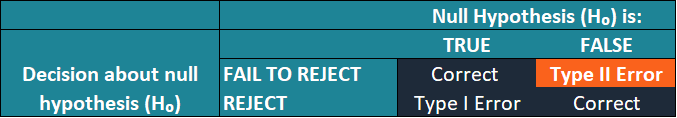# Type II Error

"False negative" error

## What is a Type II Error?

In statistical hypothesis testing, a type II error is a situation wherein a hypothesis test fails to reject the null hypothesis that is false. In other words, it causes the user to erroneously not to reject the false null hypothesis because the test lacks the statistical power to detect sufficient evidence for the alternative hypothesis. The type II error is also known as a false negative.The type II error features an inverse relationship with the power of a statistical test. It means that the higher power of a statistical test leads to the lower probability of committing a type II error. Due to such reason, the rate of a type II error (i.e., the probability of a type II error) is measured by beta (β) while the statistical power is measured by 1- β.

### How to Avoid the Type II Error?

Similar to the type I error, it is not possible to completely eliminate the type II error from a hypothesis test. The only available option is to minimize the probability of committing this type of statistical error. Since type II error is closely related to the power of a statistical test, the probability of the occurrence of the error can be minimized by increasing the power of the test.

#### 1. Increase the sample size

One of the simplest methods to increase the power of the test is to increase the sample size used in a test. The sample size primarily detects the amount of sampling error, which translates into the ability to detect the differences in a hypothesis test. The bigger sample size increases the chances to capture the differences in the statistical tests, as well as the power of a test.

#### 2. Increase the significance level

Another method is to choose the higher level of significance. For instance, a researcher may choose a significance level of 0.10 instead of the commonly acceptable 0.05 level. The higher significance level implies a higher probability of rejecting the null hypothesis when it is true.

The larger probability of rejecting the null hypothesis decreases the probability of committing a type II error while the probability of committing a type I error increases. Thus, the user should always assess the impact of type I and type II errors on their decision based on the results of a test and determine the appropriate level of statistical significance.

### Example of Type II Error

Sam is a financial analyst. He runs a hypothesis test to discover whether there is a difference in the average prices for large-cap and small-cap stocks.

In the test, Sam assumes as the null hypothesis is that there is no difference in the average prices between the large-cap and small-cap stocks. Thus, his alternative hypothesis states that a difference between the average prices does exist.

For the significance level, Sam chooses 5%. It means that there is a 5% probability that his test will reject the null hypothesis when it is actually true.

If Sam’s test incurs a type II error, then the results of the test will indicate that there is no difference in the average prices between the large-cap and small-cap stocks. However, in reality, a difference in the average prices exists.

### More Resources

CFI is the official provider of the global Financial Modeling & Valuation Analyst (FMVA)™ certification program, designed to help anyone become a world-class financial analyst. To keep advancing your career, the additional resources below will be useful:

• Type I Error
• Conditional Probability
• Framing Bias
• Mutually Exclusive Events

### Financial Analyst Certification

Become a certified Financial Modeling and Valuation Analyst (FMVA)® by completing CFI’s online financial modeling classes and training program!